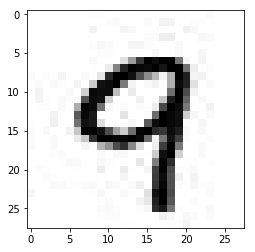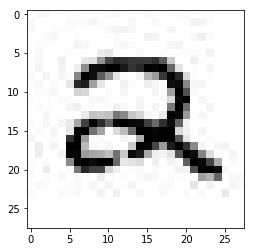Deep Learning Models -- A collection of various deep learning architectures, models, and tips for TensorFlow and PyTorch in Jupyter Notebooks.

In :
%load_ext watermark
%watermark -a 'Sebastian Raschka' -v -p tensorflow,numpy

Sebastian Raschka

CPython 3.6.1
IPython 6.1.0

tensorflow 1.1.0
numpy 1.12.1


# Using Input Pipelines to Read Data from TFRecords Files¶

TensorFlow provides users with multiple options for providing data to the model. One of the probably most common methods is to define placeholders in the TensorFlow graph and feed the data from the current Python session into the TensorFlow Session using the feed_dict parameter. Using this approach, a large dataset that does not fit into memory is most conveniently and efficiently stored using NumPy archives as explained in Chunking an Image Dataset for Minibatch Training using NumPy NPZ Archives or HDF5 data base files (Storing an Image Dataset for Minibatch Training using HDF5).

Another approach, which is often preferred when it comes to computational efficiency, is to do the "data loading" directly in the graph using input queues from so-called TFRecords files, which will be illustrated in this notebook.

Beyond the examples in this notebook, you are encouraged to read more in TensorFlow's "Reading Data" guide.

## 0. The Dataset¶

Let's pretend we have a directory of images containing two subdirectories with images for training, validation, and testing. The following function will create such a dataset of images in JPEG format locally for demonstration purposes.

In :
# Note that executing the following code
# and save all the 60,000 images as separate JPEG
# files. This might take a few minutes depending

import numpy as np

import sys
sys.path.insert(0, '..')
from helper import mnist_export_to_jpg

np.random.seed(123)
mnist_export_to_jpg(path='./')

Extracting ./train-images-idx3-ubyte.gz
Extracting ./train-labels-idx1-ubyte.gz
Extracting ./t10k-images-idx3-ubyte.gz
Extracting ./t10k-labels-idx1-ubyte.gz


The mnist_export_to_jpg function called above creates 3 directories, mnist_train, mnist_test, and mnist_validation. Note that the names of the subdirectories correspond directly to the class label of the images that are stored under it:

In :
import os

for i in ('train', 'valid', 'test'):
dirs = [d for d in os.listdir('mnist_%s' % i) if not d.startswith('.')]
print('mnist_%s subdirectories' % i, dirs)

mnist_train subdirectories ['0', '1', '2', '3', '4', '5', '6', '7', '8', '9']
mnist_valid subdirectories ['0', '1', '2', '3', '4', '5', '6', '7', '8', '9']
mnist_test subdirectories ['0', '1', '2', '3', '4', '5', '6', '7', '8', '9']


To make sure that the images look okay, the snippet below plots an example image from the subdirectory mnist_train/9/:

In :
%matplotlib inline
import matplotlib.image as mpimg
import matplotlib.pyplot as plt
import os

some_img = os.path.join('./mnist_train/9/', os.listdir('./mnist_train/9/'))

print(img.shape)
plt.imshow(img, cmap='binary');

(28, 28)Note: The JPEG format introduces a few artifacts that we can see in the image above. In this case, we use JPEG instead of PNG. Here, JPEG is used for demonstration purposes since that's still format many image datasets are stored in.

## 1. Saving images as TFRecords files¶

First, we are going to convert the images into a binary TFRecords file, which is based on Google's protocol buffer format:

The recommended format for TensorFlow is a TFRecords file containing tf.train.Example protocol buffers (which contain Features as a field). You write a little program that gets your data, stuffs it in an Example protocol buffer, serializes the protocol buffer to a string, and then writes the string to a TFRecords file using the tf.python_io.TFRecordWriter. For example, tensorflow/examples/how_tos/reading_data/convert_to_records.py converts MNIST data to this format.

In :
import glob
import numpy as np
import tensorflow as tf

def images_to_tfrecords(data_stempath='./mnist_',
shuffle=False,
random_seed=None):

def int64_to_feature(value):
return tf.train.Feature(int64_list=tf.train.Int64List(value=value))

for s in ['train', 'valid', 'test']:

with tf.python_io.TFRecordWriter('mnist_%s.tfrecords' % s) as writer:

img_paths = np.array([p for p in glob.iglob('%s%s/**/*.jpg' %
(data_stempath, s),
recursive=True)])

if shuffle:
rng = np.random.RandomState(random_seed)
rng.shuffle(img_paths)

for idx, path in enumerate(img_paths):
label = int(os.path.basename(os.path.dirname(path)))
image = image.reshape(-1).tolist()

example = tf.train.Example(features=tf.train.Features(feature={
'image': int64_to_feature(image),
'label': int64_to_feature([label])}))

writer.write(example.SerializeToString())


Note that it is important to shuffle the dataset so that we can later make use of TensorFlow's tf.train.shuffle_batch function and don't need to load the whole dataset into memory to shuffle epochs.

In :
images_to_tfrecords(shuffle=True, random_seed=123)


Just to make sure that the images were serialized correctly, let us load an image back from TFRecords using the tf.python_io.tf_record_iterator and display it:

In :
import tensorflow as tf
import numpy as np

record_iterator = tf.python_io.tf_record_iterator(path='mnist_train.tfrecords')

for r in record_iterator:
example = tf.train.Example()
example.ParseFromString(r)

label = example.features.feature['label'].int64_list.value
print('Label:', label)
img = np.array(example.features.feature['image'].int64_list.value)
img = img.reshape((28, 28))
plt.imshow(img, cmap='binary')
plt.show
break

Label: 2So far so good, the image above looks okay. In the next secction, we will introduce a slightly different approach for loading the images, namely, the TFRecordReader, which we need to load images inside a TensorFlow graph.

Roughly speaking, we can regard the TFRecordReader as a class that let's us load images "symbolically" inside a TensorFlow graph. A TFRecordReader uses the state in the graph to remember the location of a .tfrecord file that it reads and lets us iterate over training examples and batches after initializing the graph as we will see later.

To see how it works, let's start with a simple function that reads one image at a time:

In :
def read_one_image(tfrecords_queue, normalize=True):

features = tf.parse_single_example(value,
features={'label': tf.FixedLenFeature([], tf.int64),
'image': tf.FixedLenFeature(, tf.int64)})
label = tf.cast(features['label'], tf.int32)
image = tf.cast(features['image'], tf.float32)
onehot_label = tf.one_hot(indices=label, depth=10)

if normalize:
# normalize to [0, 1] range
image = image / 255.

return onehot_label, image


To use this read_one_image function to fetch images in a TensorFlow session, we will make use of queue runners as illustrated in the following example:

In :
g = tf.Graph()
with g.as_default():

queue = tf.train.string_input_producer(['mnist_train.tfrecords'],
num_epochs=10)

with tf.Session(graph=g) as sess:
sess.run(tf.local_variables_initializer())
sess.run(tf.global_variables_initializer())

coord = tf.train.Coordinator()

for i in range(10):
one_label, one_image = sess.run([label, image])

print('Label:', one_label, '\nImage dimensions:', one_image.shape)

coord.request_stop()

Label: [ 0.  0.  0.  0.  0.  0.  0.  1.  0.  0.]
Image dimensions: (784,)

• The tf.train.string_input_producer produces a filename queue that we iterate over in the session. Note that we need to call sess.run(tf.local_variables_initializer()) if we define a fixed number of num_epochs in tf.train.string_input_producer. Alternatively, num_epochs can be set to None to iterate "infinitely."

• The tf.train.start_queue_runners function uses a queue runner that uses a separate thread to load the filenames from the queue that we defined in the graph without blocking the reader.

However, we rarely (want to) train neural networks with one datapoint at a time but use minibatches instead. TensorFlow also has some really convenient utility functions to do the batching conveniently. In the following code example, we will use the tf.train.shuffle_batch function to load the images and labels in batches of size 64:

In :
g = tf.Graph()
with g.as_default():

queue = tf.train.string_input_producer(['mnist_train.tfrecords'],
num_epochs=10)

label_batch, image_batch = tf.train.shuffle_batch([label, image],
batch_size=64,
capacity=5000,
min_after_dequeue=2000,
seed=123)

with tf.Session(graph=g) as sess:
sess.run(tf.local_variables_initializer())
sess.run(tf.global_variables_initializer())

coord = tf.train.Coordinator()

for i in range(10):
many_labels, many_images = sess.run([label_batch, image_batch])

print('Batch size:', many_labels.shape)

coord.request_stop()

Batch size: 64


The other relevant arguments we provided to tf.train.shuffle_batch are described below:

• capacity: An integer that defines the maximum number of elements in the queue.
• min_after_dequeue: The minimum number elements in the queue after a dequeue, which is used to ensure that a minimum number of data points have been loaded for shuffling.
• num_threads: The number of threads for enqueuing.

## 3. Use queue runners to train a neural network¶

In this section, we will take the concepts that were introduced in the previous sections and train a multilayer perceptron from the 'mnist_train.tfrecords' file:

In :
##########################
### SETTINGS
##########################

# Hyperparameters
learning_rate = 0.1
batch_size = 128
n_epochs = 15
n_iter = n_epochs * (45000 // batch_size)

# Architecture
n_hidden_1 = 128
n_hidden_2 = 256
height, width = 28, 28
n_classes = 10

##########################
### GRAPH DEFINITION
##########################

g = tf.Graph()
with g.as_default():

tf.set_random_seed(123)

# Input data
queue = tf.train.string_input_producer(['mnist_train.tfrecords'],
num_epochs=None)

label_batch, image_batch = tf.train.shuffle_batch([label, image],
batch_size=batch_size,
seed=123,
capacity=5000,
min_after_dequeue=2000)

tf_images = tf.placeholder_with_default(image_batch,
shape=[None, 784],
name='images')
tf_labels = tf.placeholder_with_default(label_batch,
shape=[None, 10],
name='labels')

# Model parameters
weights = {
'h1': tf.Variable(tf.truncated_normal([height*width, n_hidden_1], stddev=0.1)),
'h2': tf.Variable(tf.truncated_normal([n_hidden_1, n_hidden_2], stddev=0.1)),
'out': tf.Variable(tf.truncated_normal([n_hidden_2, n_classes], stddev=0.1))
}
biases = {
'b1': tf.Variable(tf.zeros([n_hidden_1])),
'b2': tf.Variable(tf.zeros([n_hidden_2])),
'out': tf.Variable(tf.zeros([n_classes]))
}

# Multilayer perceptron
layer_1 = tf.nn.relu(layer_1)
layer_2 = tf.nn.relu(layer_2)
out_layer = tf.matmul(layer_2, weights['out']) + biases['out']

# Loss and optimizer
loss = tf.nn.softmax_cross_entropy_with_logits(logits=out_layer, labels=tf_labels)
cost = tf.reduce_mean(loss, name='cost')
train = optimizer.minimize(cost, name='train')

# Prediction
prediction = tf.argmax(out_layer, 1, name='prediction')
correct_prediction = tf.equal(tf.argmax(label_batch, 1), tf.argmax(out_layer, 1))
accuracy = tf.reduce_mean(tf.cast(correct_prediction, tf.float32), name='accuracy')

with tf.Session(graph=g) as sess:
sess.run(tf.global_variables_initializer())
saver0 = tf.train.Saver()

coord = tf.train.Coordinator()

avg_cost = 0.
iter_per_epoch = n_iter // n_epochs
epoch = 0

for i in range(n_iter):
_, cost = sess.run(['train', 'cost:0'])
avg_cost += cost

if not i % iter_per_epoch:
epoch += 1
avg_cost /= iter_per_epoch
print("Epoch: %03d | AvgCost: %.3f" % (epoch, avg_cost))
avg_cost = 0.

coord.request_stop()

saver0.save(sess, save_path='./mlp')

Epoch: 001 | AvgCost: 0.007
Epoch: 002 | AvgCost: 0.469
Epoch: 003 | AvgCost: 0.240
Epoch: 004 | AvgCost: 0.183
Epoch: 005 | AvgCost: 0.151
Epoch: 006 | AvgCost: 0.128
Epoch: 007 | AvgCost: 0.110
Epoch: 008 | AvgCost: 0.099
Epoch: 009 | AvgCost: 0.087
Epoch: 010 | AvgCost: 0.078
Epoch: 011 | AvgCost: 0.070
Epoch: 012 | AvgCost: 0.063
Epoch: 013 | AvgCost: 0.058
Epoch: 014 | AvgCost: 0.051
Epoch: 015 | AvgCost: 0.047


After looking at the graph above, you probably wondered why we used tf.placeholder_with_default to define the two placeholders:

tf_images = tf.placeholder_with_default(image_batch,
shape=[None, 784],
name='images')
tf_labels = tf.placeholder_with_default(label_batch,
shape=[None, 10],
name='labels')


In the training session above, these placeholders are being ignored if we don't feed them via a session's feed_dict, or in other words "[A tf.placeholder_with_default is a] placeholder op that passes through input when its output is not fed" (https://www.tensorflow.org/api_docs/python/tf/placeholder_with_default).

However, these placeholders are useful if we want to feed new data to the graph and make predictions after training as in a real-world application, which we will see in the next section.

## 4. Feeding new datapoints through placeholders¶

To demonstrate how we can feed new data points to the network that are not part of the mnist_train.tfrecords file, let's use the test dataset and load the images into Python and pass it to the graph using a feed_dict:

In :
record_iterator = tf.python_io.tf_record_iterator(path='mnist_test.tfrecords')

with tf.Session() as sess:

saver1 = tf.train.import_meta_graph('./mlp.meta')
saver1.restore(sess, save_path='./mlp')

num_correct = 0
for idx, r in enumerate(record_iterator):
example = tf.train.Example()
example.ParseFromString(r)
label = example.features.feature['label'].int64_list.value
image = np.array(example.features.feature['image'].int64_list.value)

pred = sess.run('prediction:0',
feed_dict={'images:0': image.reshape(1, 784)})

num_correct += int(label == pred)
acc = num_correct / (idx + 1) * 100

print('Test accuracy: %.1f%%' % acc)

INFO:tensorflow:Restoring parameters from ./mlp
Test accuracy: 97.3%